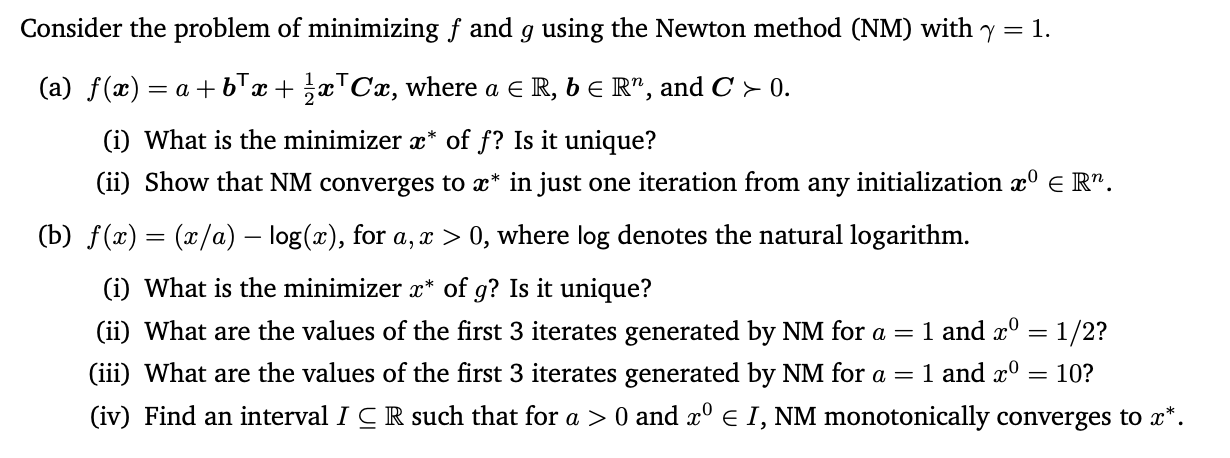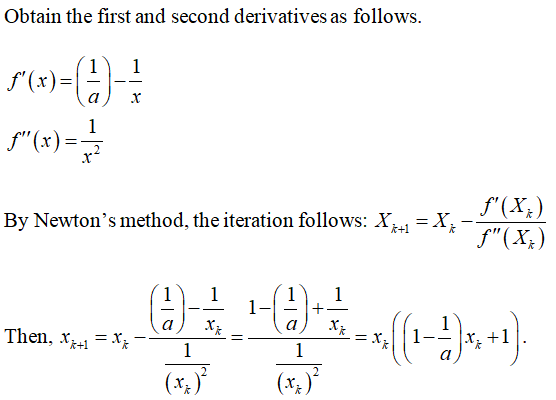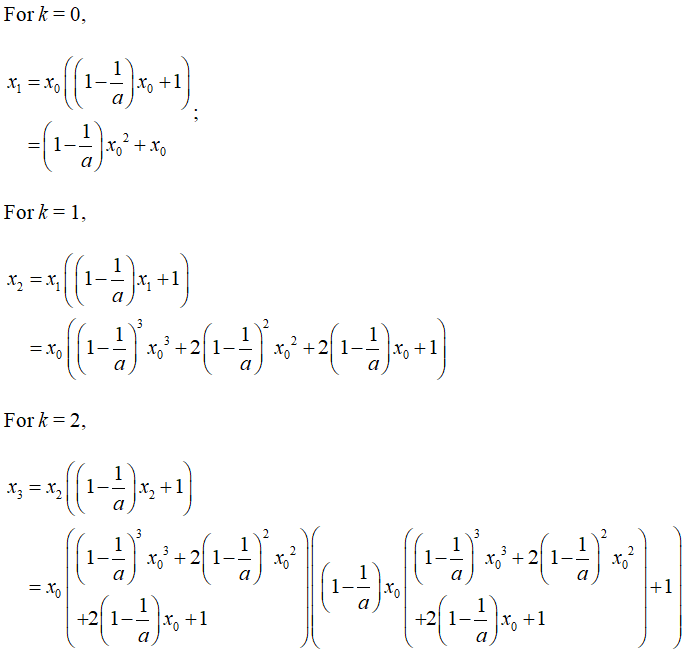# Consider the problem of minimizing f and g using the Newton method (NM) with y = 1.(a) f(x) := a + bTx + x™Cx, where a E R, b E R", and C > 0.(i) What is the minimizer x* of f? Is it unique?(ii) Show that NM converges to x* in just one iteration from any initialization x° e R".(b) f(x) = (x/a) – log(x), for a, x > 0, where log denotes the natural logarithm.(i) What is the minimizer x* of g? Is it unique?1 and x° = 1/2?1 and x°(ii) What are the values of the first 3 iterates generated by NM for a =(iii) What are the values of the first 3 iterates generated by NM for a == 10?(iv) Find an interval I C R such that for a > 0 and x° e I, NM monotonically converges to x*.

Question
6 viewshelp_outlineImage TranscriptioncloseConsider the problem of minimizing f and g using the Newton method (NM) with y = 1. (a) f(x) : = a + bTx + x™Cx, where a E R, b E R", and C > 0. (i) What is the minimizer x* of f? Is it unique? (ii) Show that NM converges to x* in just one iteration from any initialization x° e R". (b) f(x) = (x/a) – log(x), for a, x > 0, where log denotes the natural logarithm. (i) What is the minimizer x* of g? Is it unique? 1 and x° = 1/2? 1 and x° (ii) What are the values of the first 3 iterates generated by NM for a = (iii) What are the values of the first 3 iterates generated by NM for a = = 10? (iv) Find an interval I C R such that for a > 0 and x° e I, NM monotonically converges to x*. fullscreen
check_circle

Note:

The first three questions of part (b) are answered, kindly post the remaining part separately.

(i) What is the minimizer x* of g? Is it unique?Substitution:...

### Want to see the full answer?

See Solution

#### Want to see this answer and more?

Solutions are written by subject experts who are available 24/7. Questions are typically answered within 1 hour.*

See Solution
*Response times may vary by subject and question.
Tagged in